Select Page

# Maths 12 Science Probability CBSE Answers for MCQ in English

Maths 12 Science Probability CBSE Answers for MCQ in English to enable students to get Answers in a narrative video format for the specific question.

Expert Teacher provides Maths 12 Science Probability CBSE Answers for MCQ through Video Answers in English language. This video solution will be useful for students to understand how to write an answer in exam in order to score more marks. This teacher uses a narrative style for a question from Probability not only to explain the proper method of answering question, but deriving right answer too.

Please find the question below and view the Answer in a narrative video format.

Question:

## Similar Questions from CBSE, 12th Science, Maths, Probability

Question 1 : A bag A contains 4 black and 6 red balls and bag B 7 black and 3 red balls. A die is thrown. If 1 or 2 appears on it, then bag A is chosen, otherwise bag B. It two balls are drawn at random (without replacement) from the selected bag, find the probability of one of them being red and another black.        (View Answer Video)

Question 2 : One card is drawn from a pack of 52 cards. Find the probability of getting a black face card: (View Answer Video)

Question 3 : An experiment succeeds thrice as often as it fails. Find the probability that in the next trials, there will be atleast 3 successes.    (View Answer Video)

Question 4 : A die is thrown 6 times. If 'getting an odd number' is a 'success', what is the probability of obtaining:
atleast 5 successes?

Question 5 :  Probability of solving specific problem independently by A and B are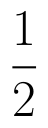and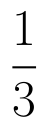respectively. If both try to solve the problem, independently, then find the probability that exactly one of them solves the problem.  (View Answer Video)

### Vector Algebra

Question 1 : Find the unit vector in the direction of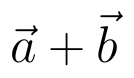if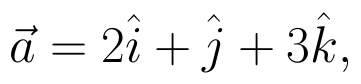and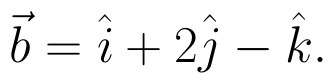(View Answer Video)

Question 2 :  Write the value of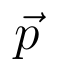for which the vectors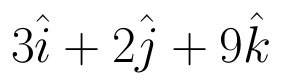and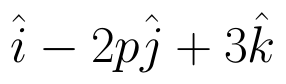are parallel vectors.  (View Answer Video)

Question 3 : Find the value of x and y so that the vectors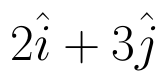and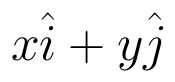are equal. (View Answer Video)

Question 4 : Compute the magnitude of the following vectors: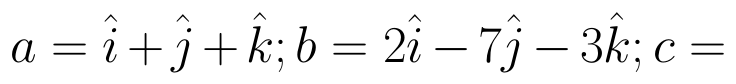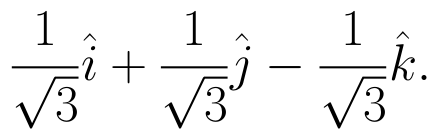(View Answer Video)

Question 5 :  Find the sum of the vectors :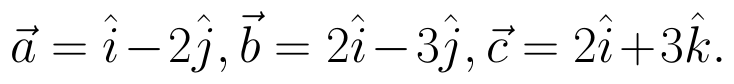(View Answer Video)

### Relations and Functions

Question 1 : Let A = {1, 2, 3}. Then, number of relations containing (1, 2) and (1, 3) which are reflexive and symmetric but not transitive is, (View Answer Video)

Question 2 : The law a + b = b + a is called ______. (View Answer Video)

Question 3 : If f is the greatest integer function and g is the modulus function . Write the value of g o f(-1/3) – f o g ( -1/3 ). (View Answer Video)

Question 4 : A functiondefined as f(x) = 5x is, (View Answer Video)

Question 5 : If the mapping f and g are given by f = {(1, 2), (3, 5), (4, 1)} and g = {(2, 3), (5, 1), (1, 3)}, Find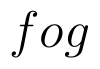. (View Answer Video)

### Application of Derivatives

Question 1 : A cylindrical tank of radius 10 m is being filled with wheat at the rate of the 314 cubic meters per hour. Then the depth of the wheat is increasing at the rate of ___________. (View Answer Video)

Question 2 : The line y = mx + 1 is a tangent to the curve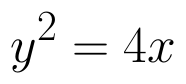if the value of m is: (View Answer Video)

Question 3 : The normal to the curve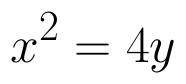passing (1, 2) is: (View Answer Video)

Question 4 : Find two positive numbers x  and y such that x+y=60 and is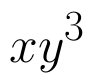maximum. (View Answer Video)

Question 5 : The total revenue (in Rs) received from the sale of 'x' units of a product is given by :
R(x) =3x2+36x+5.
FInd the marginal revenue when x=5, where by marginal revenue we mean the rate of change of total revenue with respect to the number of items sold at an instant. (View Answer Video)